International
Tables for
Crystallography
Volume B
Reciprocal space
Edited by U. Shmueli

International Tables for Crystallography (2006). Vol. B. ch. 2.5, pp. 308-309   | 1 | 2 |

## Section 2.5.4.3. Intensities of diffraction beams

B. K. Vainshteinc and B. B. Zvyagind

#### 2.5.4.3. Intensities of diffraction beams

| top | pdf |

The intensities of scattering by a crystal are determined by the scattering amplitudes of atoms in the crystal, given by (see also Section 5.2.1)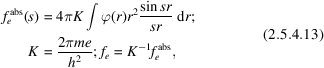where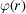is the potential of an atom and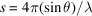. The absolute values of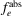have the dimensionality of length L. In EDSA it is convenient to use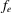without K. The dimensionality ofis [potential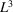]. With the expression ofin V Å3 the value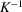in (2.5.4.13)is 47.87 V Å2.

The scattering atomic amplitudes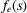differ from the respective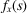X-ray values in the following: while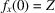(electron shell charge), the atomic amplitude at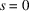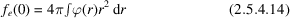is the full potential' of the atom. On average,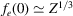, but for small atomic numbers Z, owing to the peculiarities in the filling of the electron shells,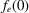exhibits within periods of the periodic table of elements reverse motion', i.e. they decrease with Z increasing (Vainshtein, 1952, 1964). At large,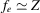. The atomic amplitudes and, consequently, the reflection intensities, are recorded, in practice, up to values of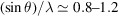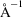, i.e. up to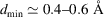.

The structure amplitude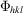of a crystal is determined by the Fourier integral of the unit-cell potential (see Chapter 1.2),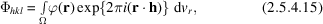where Ω is the unit-cell volume. The potential of the unit cell can be expressed by the potentials of the atoms of which it is composed: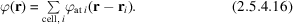The thermal motion of atoms in a crystal is taken into account by the convolution of the potential of an atom at rest with the probability function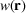describing the thermal motion: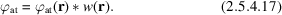Accordingly, the atomic temperature factor of the atom in a crystal is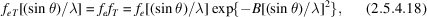where the Debye temperature factor is written for the case of isotropic thermal vibrations. Consequently, the structure amplitude is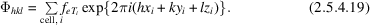This general expression is transformed (see IT I, 1952) according to the space group of a given crystal.

To determine the structure amplitudes in EDSA experimentally, one has to use specimens satisfying the kinematic scattering condition, i.e. those consisting of extremely thin crystallites. The limit of the applicability of the kinematic approximation (Blackman, 1939; Vainshtein, 1964) can be estimated from the formula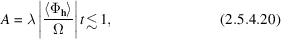where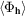is the averaged absolute value of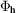(see also Section 5.2.1). Sinceare proportional to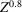, condition (2.5.4.20)is better fulfilled for crystals with light and medium atoms. Condition (2.5.4.20)is usually satisfied for textured and polycrystalline specimens. But for mosaic single crystals as well, the kinematic approximation limit is, in view of their real structure, substantially wider than estimated by (2.5.4.20)for ideal crystals. The fulfillment of the kinematic law for scattering can be, to a greater or lesser extent, estimated by comparing the decrease of experimental intensity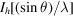averaged over definite angular intervals, and sums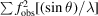calculated for the same angular intervals.

For mosaic single-crystal films the integral intensity of reflection is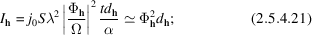for textures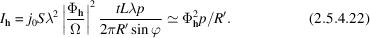Here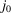is the incident electron-beam density, S is the irradiated specimen area, t is the thickness of the specimen, α is the average angular spread of mosaic blocks, R′ is the horizontal coordinate of the reflection in the diffraction pattern and p is the multiplicity factor. In the case of polycrystalline specimens the local intensity in the maximum of the ring reflection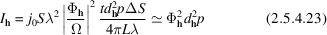is measured, where ΔS is the measured area of the ring.

The transition from kinematic to dynamic scattering occurs at critical thicknesses of crystals when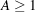(2.5.4.20). Mosaic or polycrystalline specimens then result in an uneven contribution of various crystallites to the intensity of the reflections. It is possible to introduce corrections to the experimental structure amplitudes of the first strong reflections most influenced by dynamic scattering by applying in simple cases the two-wave approximation (Blackman, 1939) or by taking into account multibeam theories (Fujimoto, 1959; Cowley, 1981; Avilov et al. 1984; see also Chapter 5.2).

The application of kinematic scattering formulae to specimens of thin crystals (5–20 nm) or dynamic corrections to thicker specimens (20–50 nm) permits one to obtain reliability factors between the calculated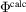and observed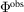structure amplitudes of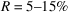, which is sufficient for structural determinations.

With the use of electron diffractometry techniques, reliability factors as small as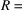2–3% have been reached and more detailed data on the distribution of the inner-crystalline potential field have been obtained, characterizing the state and bonds of atoms, including hydrogen (Zhukhlistov et al., 1997, 1998; Avilov et al., 1999).

The applicability of kinematics formulae becomes poorer in the case of structures with many heavy atoms for which the atomic amplitudes also contain an imaginary component (Shoemaker & Glauber, 1952). The experimental intensity measurement is made by a photo method or by direct recording (Avilov, 1979). In some cases the amplitudescan be determined from dynamic scattering patterns – the bands of equal thickness from a wedge-shaped crystal (Cowley, 1981), or from rocking curves.

### ReferencesInternational Tables for X-ray Crystallography (1952). Vol. I. Symmetry groups. Birmingham: Kynoch Press. (Present distributor Kluwer Academic Publishers, Dordrecht.)Google ScholarAvilov, A. S. (1979). Electrical measurement of reflection intensities on electron diffraction from mosaic single crystals. Sov. Phys. Crystallogr. 24, 103–104.Google ScholarAvilov, A. S., Kuligin, A. K., Pietsch, U., Spence, J. C. H., Tsirelson, V. G.& Zuo, J. M. (1999). Scanning system for high-energy electron diffractometry. J. Appl. Cryst. 32, 1033–1038.Google ScholarAvilov, A. S., Parmon, V. S., Semiletov, S. A. & Sirota, M. I. (1984). Calculation of reflected intensities in multiple-beam diffraction of fast electrons by polycrystalline specimens. Sov. Phys. Crystallogr. 29, 5–7.Google ScholarBlackman, M. (1939). On the intensities of electron diffraction rings. Proc. R. Soc. London Ser. A, 173, 68–82.Google ScholarCowley, J. M. (1981). Diffraction physics, 2nd ed. Amsterdam: North-Holland.Google ScholarFujimoto, F. (1959). Dynamical theory of electron diffraction in Laue-case. I. General theory. J. Phys. Soc. Jpn, 14(11), 1158–1168.Google ScholarShoemaker, V. & Glauber, R. (1952). The Born approximation in electron diffraction. Nature (London), 170, 290–291.Google ScholarVainshtein, B. K. (1952). Dependence of electron scattering on the atomic number. Dokl. Akad. Nauk SSSR, 85, 1239–1242. (In Russian.)Google ScholarVainshtein, B. K. (1964). Structure analysis by electron diffraction. Oxford: Pergamon Press.Google ScholarZhukhlistov, A. P., Avilov, A. S., Ferraris, G., Zvyagin, B. B. & Plotnikov, V. P. (1997). Statistical distribution of hydrogen over three positions in the brucite Mg(OH)2 structure from electron diffractometry data. Crystallogr. Rep. 42, 774–777.Google ScholarZhukhlistov, A. P. & Zvyagin, B. B. (1998). Crystal structure of lizardite 1T from electron diffractometry data. Crystallogr. Rep. 43, 950–955.Google Scholar